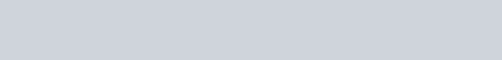# Python range()

Filed Under: Python

Python range() function is a utility function to generate the list of numbers. The list of numbers generated is useful for iteration logic.

## Python range()

If you are following our tutorial from the beginning, you may notice that many times we have used python range function.

Basically, Python range is used to generate a list of numbers. Note that, Python range function does not return a list, rather it acts like a list. The basic structure of Python range function is given below.

1. range(n) : This will generate a list of numbers from 0 to n.
2. range(a, b) : This will generate a list of numbers from a to b-1.
3. range(a, b, c) : This will generate a list of numbers from a to b-1 where step size is c.

Please remember that, the `range()` function does not return any list. In the following example, we will see that.

``````
# initialize a list from 0 to 5
init_list = [0, 1, 2, 3, 4, 5]

# it will show you the type is 'list'
print('Type of init_list is :', type(init_list))

# get the instance of range() function
instance_range = range(1, 10)

# it will show that the type is 'range'
print("Type of instance_range is :", type(instance_range))
``````

The output of the following code will be### Python range() function example

Many examples can be given for Python range function. You can use it in many places of your code. Suppose, you need to print the first 1-to-n odd numbers. You can do that easily using python range function. The code will be;

``````
# prompt for input
num = int(input('Enter the max limit: '));

# so, generate list from 1 to num(inclusive)
for i in range(1, num+1, 2):
print(i, end=' ')

``````

Here, given 11 as input, we will get the following output

``````
Enter the max limit: 11
1 3 5 7 9 11
``````

### Traversing List using Python range() for loop

However you can access python list using index of the list. In this case, the index will be generated by python range function. The following code will help you understand this clearly.

``````
# initialize a list
init_list = [1, 'abc', 23, 'def']

for i in range(len(init_list)):
print(init_list[i])

``````

The output of the following code will be

``````
1
abc
23
def
``````

So, that’s all for Python range function. Most of the time python range function is used with for loop and to iterate the list.

You can checkout complete python script and more Python examples from our GitHub Repository.

Reference: Official Documentation

close
Generic selectors
Exact matches only
Search in title
Search in content
Post Type Selectors